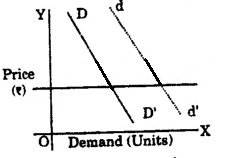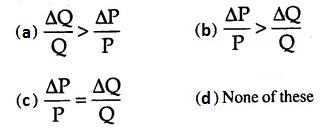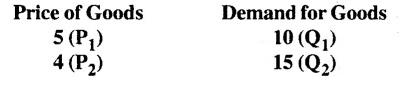# MCQ Questions for Class 12 Economics Chapter 2 Theory of Consumer Behaviour with Answers

## Theory of Consumer Behaviour Class 12 MCQs Questions with Answers

Question 1.
Who gave the cardinal concept of utility?
(a) Marshall
(b) Pigou
(c) Hicks
(d) Samuelson

Question 2.
Consumer’s behaviour is studied in:
(a) Micro Economics
(b) Macro Economics
(c) Income Analysis
(d) None of these

Question 3.
Which of the following statement is true ?
(a) Utility means want-satisfying power
(b) Utility is a function of intensity of desire
(c) Desire of consumption gives birth to utility
(d) All of these

Question 4.
Which is the First Law of Gossen?
(a) Law of Demand
(b) Law of Diminishing Marginal Utility
(c) Law of Equi-marginal Utility
(d) Consumer’s Surplus

Answer: (b) Law of Diminishing Marginal Utility

Question 5.
Which of the following is a characteristic of utility ?
(a) Utility is a psychological phenomenon
(b) Utility is subjective
(c) Utility is a relative concept
(d) All of these

Question 6.
How we calculate marginal utility ?
(a) ∆TU/∆Q
(b) ∆MU/∆Q
(c) ∆Q/∆TU
(d) ∆Q/∆MU

Question 7.
When TU becomes maximum, MU is:
(a) Positive
(b) Negative
(c) Zero
(d) None of these

Question 8.
Which of the following is true ?
(a) TU increases till MU is positive
(b) TU is maximum when MU is equal to zero
(c) TU declines when MU is negative
(d) All of these

Question 9.
Who basically propounded the concept of Law of Equimarginal Utility ?
(a) Marshall
(b) Gossen
(c) Ricardo
(d) J. S. Mill

Question 10.
In difference curve is:
(a) Convex to the origin
(b) Concave to the origin
(c) Both (a) and (b) true
(d) All of these false

Answer: (a) Convex to the origin

Question 11.
The ability of satisfying human want in a goods is called its:
(a) Productivity
(b) Satisfaction
(c) Utility
(d) Profitability

Question 12.
Slope of budget line or price line is:
(a) –$$\frac{P_x}{P_y}$$
(b) –$$\frac{P_y}{P_x}$$
(c) +$$\frac{P_x}{P_y}$$
(d) +$$\frac{P_y}{P_x}$$

Answer: (a) –$$\frac{P_x}{P_y}$$

Question 13.
Utility is related to:
(a) Usefulness
(b) Morality
(c) Satisfaction of human wants
(d) All the above

Question 14.
Utility can be measured by:
(a) Money
(b) Exchange of goods
(c) Weight of the good
(d) None of these

Question 15.
Law of Equi-marginal utility is called:
(a) Law of increasing utility
(b) Law of diminishing utility
(c) Law of substitution
(d) None of these

Question 16.
Indifference curve slopes:
(a) From right to left
(b) From left to right
(c) Both (a) and (b)
(d) None of these

Answer: (b) From left to right

Question 17.
The addition of utilities obtained from all units of a goods is called :
(a) Marginal Utility
(b) Total Utility
(c) Maximum Satisfaction

Question 18.
Who propounded the ordinal utility theory’ ?
(a) Marshall
(b) Pigou
(c) Hicks and Allen
(d) Ricardo

Question 19.
The propounder of law of diminishing marginal utility is:
(a) Gossen
(c) Chapman
(d) Hicks

Question 20.
Consumer’s equilibrium takes at a point where:
(a) MU = Price
(b) MU < Price
(c) MU > Price
(d) None of these

Question 21.
The capability of a commodity to satisfy human wants is:
(a) Consumption
(b) Utility
(c) Quality
(d) Taste

Question 22.
For the maximum satisfaction of consumer:
(a) Marginal utility of a good should be equal to its price.
(b) Marginal utility of a good should be greater than its price.
(c) There is no relation between marginal utility and price.
(d) None of these

Answer: (a) Marginal utility of a good should be equal to its price.

Question 23.
When marginal utility is negative, then total utility:
(a) is maximum
(b) Starts decreasing
(c) increases at decreasing rate
(d) None of these

Question 24.
According to the law of equi-marginal utility, the condition for consumer’s equilibrium is:
(a) $$\frac{MU_A}{P_A}$$
(b) $$\frac{MU_B}{P_B}$$
(c) Both (a) and (b)
(d) Undefined

Answer: (c) Both (a) and (b)

Question 25.
According to Marshall, utility of a commodity:
(a) Can be measured by money
(b) Cannot be measured by money
(c) Can be measured in cardinal numbers
(c) Both (a) and (b)

Answer: (c) Both (a) and (b)

Question 26.
Which element is essential for demand ?
(a) Desire to consume
(c) Willingness to consume
(d) All of these

Question 27.
Demand Curve generally slopes:
(a) Upward from left to right
(b) Downward from left to right
(c) Parallel to X-axis
(d) Parallel to Y-axis

Answer: (b) Downward from left to right

Question 28.
In which goods, price fall does not make any increase in demand ?
(a) Necessities Goods
(b) Comfort Goods
(c) Luxuries Goods
(d) None of these

Question 29.
Which of the following factor affects demand ?
(a) Price
(b) Change in income
(c) Taste of the Consumer
(d) All of these

Question 30.
Goods, which can alternatively be used, are called:
(a) Complementary Goods
(b) Substitutes
(c) Comforts
(d) None of these

Question 31.
Law of Demand is a:
(a) Qualitative Statement
(b) Quantitative Statement
(c) Both (a) and (b)
(d) None of these

Question 32.
Which of the following is a demand function ?
(a) PX
(b) DX = PX
(c) Dx = (Px)
(d) None of these

Question 33.
When change in the price of goods-X affects the demand of goods-Y, this demand is called:
(a) Price Demand
(b) Income Demand
(c) Cross Demand
(d) All of these

Question 34.
For normal goods, Law of Demand states the relationship between price and quantity of goods:
(a) Direct
(b) Positive
(c) Indirect
(d) None of the above

Question 35.
Which of the following is a reason for fall in demand ?
(a) Fall in Income
(b) Fall in Number of Buyers
(c) Fall in Taste of Consumer
(d) All the above

Question 36.
With rise in coffee price, the demand of tea:
(a) Rises
(b) Falls
(c) Remains stable
(d) None of these

Question 37.
Contraction in demand appears when:
(a) Price rises and demand falls
(b) Price rises and demand also rises
(c) Price remains stable and demand falls
(d) Price falls but demand remains stable

Answer: (a) Price rises and demand falls

Question 38.
Which is a reason of change in demand ?
(a) Change in consumer’s income
(b) Change in price of related goods
(c) Population increase
(d) All pf these

Question 39.
For a change in which of the following, there is no change in demand ?
(a) Change in price
(b) Change in income
(c) Change in taste and fashion
(d) None of these

Question 40.
With a rise in price the demand for ‘Giffin’ goods:
(a) increases
(b) decreases
(c) remains constant
(d) becomes unstable

Question 41.
Hie slope of the demand Curve of a normal goods is:
(a) Negative
(b) Positive
(c) Zero
(d) Undefined

Question 42.
With an increase in income consumer decreases the consumption of which goods ?
(a) Inferior goods
(b) Normal goods
(c) Giffin goods
(d) Both (a) and (b)

Question 43.
The demand curve of a good shifts from DD’ to dd(a) fail in the price of the goods
(b) rise in the price of the goods
(c) rise in the price of substitute goods
(d) rise in the price of complementary goods

Answer: (c) rise in the price of substitute goods

Question 44.
Elasticity of demand is a:
(a) Qualitative Statement
(b) Quantitative Statement
(c) Both (a) and (b)
(d) None of the above

Question 45.
Which of the following is a formula for measuring the elasticity of demand ?Question 46.
For Giffin goods, price elasticity of demand is :
(a) Negative
(b) Positive
(c) Zero
(d) None of these

Question 47.
Following figure shows:(a) High Elastic Demand
(b) Perfectly Elastic Demand
(c) Perfectly Inelastic Demand
(d) Inelastic Demand

Question 48.
Which of the following shows elasticity less than one ?
(a) Necessity Goods
(b) Comforts
(c) Luxuries
(d) All of these

Question 49.
With which method, elasticity of demand is measured ?
(a) Total Expenditure Method .
(b) Percentage or Proportionate Method
(c) Point Method
(d) All of these

Question 50.
Elastic demand is shown by:Answer: (a) $$\frac{ΔQ}{Q} > \frac{ΔP}{P}$$

Question 51.
What is the price elasticity in following example ?(a) -2.5
(b) + 3.5
(c) + 4.0
(d) None of these

Question 52.
Who propounded the percentage or proportionate method of measuring elasticity of demand ?
(a) Marshall
(b) Flux
(c) Hicks
(d) None of these

Question 53.
Which of the following factor affects elasticity of demand ?
(a) Nature of Goods
(b) Price Level
(c) Income Level
(d) All of these

Question 54.
How many types elasticity of uemand has ?
(a) Three
(b) Five
(c)Six
(d) Seven

Question 55.
Elasticity of demand for necessities is :
(a) Zero
(b) Unlimited
(c) Greater than unity
(d) Less than unity

Question 56.
Price elasticity of demand means :
(a) Change in demand due to change in price
(b) Change in demand
(c) Change in real income
(d) Change in Price

Answer: (a) Change in demand due to change in price

Question 57.
The elasticity of demand at the mid-point of a straight line demand curve:
(a) will be zero
(b) will be unity
(c) will be infinity
(d) None of these

Question 58.
If the demand for a good changes by 60% due to 40% change in price, the elasticity of demand is :
(a) 0.5
(b) -1.5
(c) 1
(d) zero

Question 59.
For luxury goods the demand is:
(a) Inelastic
(b) Elastic
(c) Highly elastic
(d) Perfectly Inelastic

Question 60.
Any statement about demand for a good is considered complete only when the following is/are mentioned in:
(a) Price of the good
(b) Quantity of the good
(c) Period of time
(d) All of these

Answer: (a) Price of the good

Question 61.
Consumer is in equilibrium when:
(a) MUx = PUx
(b) MUx > PUx
(c) MUx < Px
(d) MUx ÷ Px

Question 62.
Marshall has given the law of Equimarginal utility related:
(a) Related to goods
(b) Related to money
(c) In relation to both
(d) None of these.

Question 63.
How many tremendous curves can touch the budget line:
(a) One
(b) Two
(c) Several
(d) Depends on the basis of indifference maps.

Question 64.
Indifference curves were first introduced by the English economist in 1881 by:
(a) Edge worth
(b) Pareto
(c) Myers
(d) Hicks.

Question 65.
Any statement about the demand of an object is considered complete when it is mentioned in the following:
(a) Price of good
(b) Demand of good
(c) Time period
(d) All of the above.

Answer: (d) All of the above.

Question 66.
If price of goods ‘X’ falls leading to increase in demand of goods ‘ Y’ then both the goods are:
(a) Substitute goods
(b) Complementary goods
(c) Not related
(d) Competitor.

Question 67.
According to total outlay method, the demand of a good is sinelastic when:
(a) Price will fall with the increase in amount spent
(b) When price of good decreases and money spent decreases
(c) Expenditure remains the same, even if price falls
(d) Expenditure decreases with the increase in price.

Answer: (b) When price of good decreases and money spent decreases

Fill in the blanks:

1. Consumer is a …………. human being.

2. If the price of substitute goods increases then the demand curve shifts to the ………….

3. …………. propounded the law of Diminishing Returns.

4. According to Marshall utility can be measured in terms of ………….

5. An indifference curve gives …………. level of satisfaction to the consumers.

6. Car and Petrol are goods ………….

7. There is …………. relation between price and demand.

State true or false:

1. Utility is an intensive assumption.

2. The proportion of the cost of two goods measures the slope of budget line.

3. Demand curve is generally negative sloped.

4. Budget set is a collection of all bundles that a consumer purchases from their income at market prices.

5. The elasticity of the demand of the object and the expenditure on the object is closely related.